##### Common Core Math For Parents For Dummies with Videos Online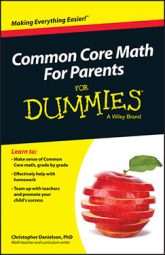In Common Core math, eighth grade is the first time students meet the term function. Mathematicians use the idea of a function to describe operations such as addition and multiplication, transformations of geometric figures, relationships between variables, and many other things.

A function is a rule for pairing things up with each other. A function has inputs, it has outputs, and it pairs the inputs with the outputs. There is one important restriction to this pairing: Each input can be paired with only one output.

An example of something that isn't a function is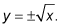In this case, the "plus or minus" sign allows for more than one y-value for the same x-value. This new rule generates these ordered pairs, for example: (4, 2) and (4, –2). The same input (4) is paired with two different outputs (2 and –2), which means that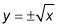isn't a function.

For example, the function y = x2 pairs numbers with each other. The x-values are the inputs and the y-values are the outputs. Often, people write the pairs using this notation: (x, y), which means that (1, 1), (2, 4), and (–3, 9) are all pairs generated by the rule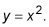As required, this function has only one output for each input. There is no requirement the other way around, though. It's okay that (2, 4) and (–2, 4) are both pairs generated by this rule. In this case, one output is paired with two different inputs, which can happen (and it often does) with a function.

Students learn to identify functions and non-functions from their graphs. If two points are on a graph so that one point is directly above the other, it means that the same x-value is paired with two different y-values, so the graph doesn't describe a function. Sometimes, it's referred to as the vertical line test.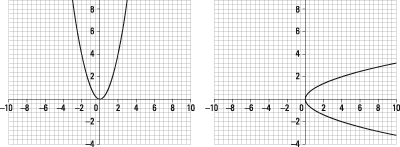On the left, a function. On the right, not a function.

The graph on the left in the figure is of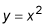and the graph on the right is ofKnowing the definition of a function is a good thing. In mathematics, definitions help you to sort the world out in a matter-of-fact way. Something either is a function or it isn't a function because it either fits the definition or it doesn't.

Most people, however, don't go through life — or even through math class — by constantly thinking about definitions. Getting better at referring to and using definitions is a Standard for Mathematical Practice, but most people refer first to a set of mental images when they think about categorizing things. People tend to ask whether something looks like a function or not before they refer to the definition. Because students spend so much time with functions such as the one on the left in the first figure, they tend to miss the strange cases of functions such as those in the next figure.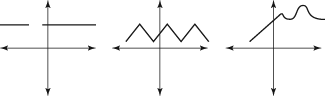Examples of functions.

Each of the graphs in this figure represents a function because it fits the definition of pairing x-values with y-values in such a way that each x-value gets only one y-value. However, none of the graphs in the figure looks like the functions that students encounter as they go about their average day in a traditional algebra class. In a Common Core classroom, students encounter (and even produce) these kinds of examples in the service of better understanding functions.